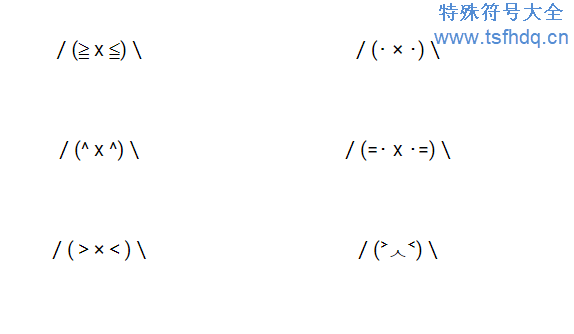# 漂亮的小兔子符号/(≧ x ≦)\

/(･ × ･)\

/(=´x`=)\

/(^ x ^)\

/(=･ x ･=)\

/(^ × ^)\

/(>×<)\

/(˃ᆺ˂)\